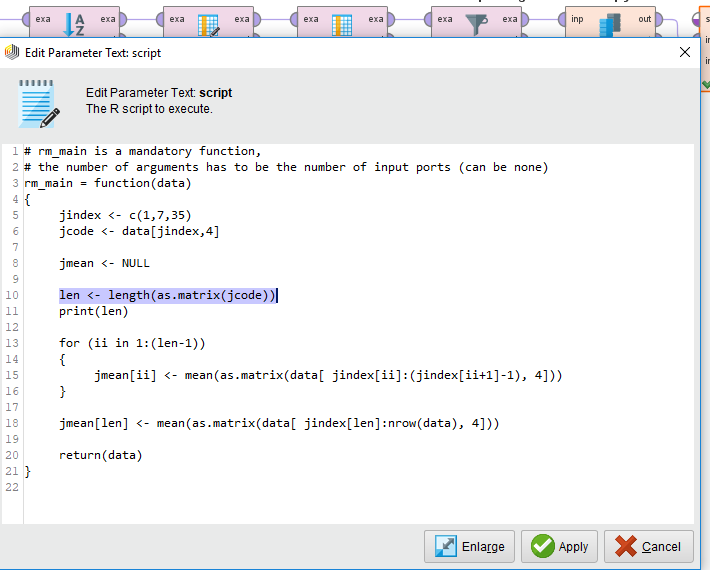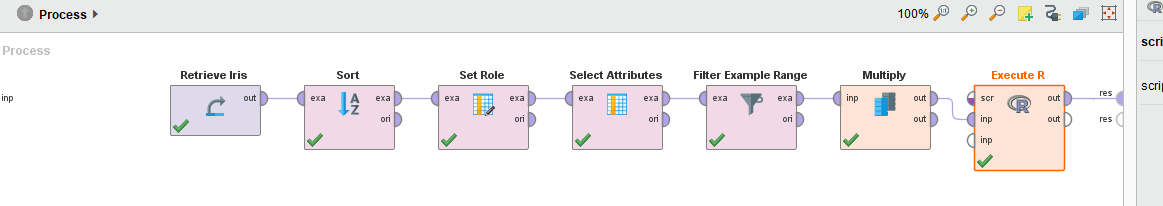# Trouble in Execute R

Member Posts: 35Contributor II
edited August 2019 in Help

Hello, everyone

While using in "Execute R", I have a trouble

I attached the process file, log message, and captured image of error message

Data I used is "Iris" data set given in  Rapidminer

Please analyze the script of R in "Execute R", which I also attached the script

whose file name is "executer.txt"

Also, I attached data file "1.csv", which I tested on R-3.6.1 and works OK in R

Thank you and have a nice day!

Tagged:

• Administrator, Employee, RapidMiner Certified Analyst, RapidMiner Certified Expert, Member Posts: 363RM Data Scientist
Hi @Rapidminerpartner,
a quick fix is to convert the data frame to matrix and get the length```<?xml version="1.0" encoding="UTF-8"?><process version="9.4.000-BETA2">
<context>
<input/>
<output/>
<macros/>
</context>
<operator activated="true" class="process" compatibility="9.4.000-BETA2" expanded="true" name="Process">
<parameter key="logverbosity" value="init"/>
<parameter key="random_seed" value="2001"/>
<parameter key="send_mail" value="never"/>
<parameter key="process_duration_for_mail" value="30"/>
<parameter key="encoding" value="SYSTEM"/>
<process expanded="true">
<operator activated="true" class="retrieve" compatibility="9.4.000-BETA2" expanded="true" height="68" name="Retrieve Iris" width="90" x="179" y="34">
<parameter key="repository_entry" value="//Samples/data/Iris"/>
</operator>
<operator activated="true" class="sort" compatibility="9.4.000-BETA2" expanded="true" height="82" name="Sort" width="90" x="313" y="34">
<parameter key="attribute_name" value="a4"/>
<parameter key="sorting_direction" value="increasing"/>
</operator>
<operator activated="true" class="set_role" compatibility="9.4.000-BETA2" expanded="true" height="82" name="Set Role" width="90" x="447" y="34">
<parameter key="attribute_name" value="id"/>
<parameter key="target_role" value="regular"/>
<parameter key="label" value="regular"/>
</list>
</operator>
<operator activated="true" class="select_attributes" compatibility="9.4.000-BETA2" expanded="true" height="82" name="Select Attributes" width="90" x="581" y="34">
<parameter key="attribute_filter_type" value="subset"/>
<parameter key="attribute" value=""/>
<parameter key="attributes" value="id|label"/>
<parameter key="use_except_expression" value="false"/>
<parameter key="value_type" value="attribute_value"/>
<parameter key="use_value_type_exception" value="false"/>
<parameter key="except_value_type" value="time"/>
<parameter key="block_type" value="attribute_block"/>
<parameter key="use_block_type_exception" value="false"/>
<parameter key="except_block_type" value="value_matrix_row_start"/>
<parameter key="invert_selection" value="true"/>
<parameter key="include_special_attributes" value="false"/>
</operator>
<operator activated="true" class="filter_example_range" compatibility="9.4.000-BETA2" expanded="true" height="82" name="Filter Example Range" width="90" x="715" y="34">
<parameter key="first_example" value="1"/>
<parameter key="last_example" value="41"/>
<parameter key="invert_filter" value="false"/>
</operator>
<operator activated="true" class="multiply" compatibility="9.4.000-BETA2" expanded="true" height="82" name="Multiply" width="90" x="849" y="34"/>
<operator activated="true" class="r_scripting:execute_r" compatibility="9.1.000" expanded="true" height="103" name="Execute R" width="90" x="983" y="34">
<parameter key="script" value="# rm_main is a mandatory function, &#10;# the number of arguments has to be the number of input ports (can be none)&#10;rm_main = function(data)&#10;{&#10;&#9;jindex &lt;- c(1,7,35)&#10;&#9;jcode &lt;- data[jindex,4]&#10;&#10;&#9;jmean &lt;- NULL&#10;&#10;&#9;len &lt;- length(as.matrix(jcode))&#10;&#9;print(len)&#10;&#10;&#9;for (ii in 1:(len-1))&#10;&#9;{&#10;&#9;&#9;jmean[ii] &lt;- mean(as.matrix(data[ jindex[ii]:(jindex[ii+1]-1), 4]))&#10;&#9;}&#10;&#10;&#9;jmean[len] &lt;- mean(as.matrix(data[ jindex[len]:nrow(data), 4]))&#10;&#10;&#9;return(data)&#10;}&#10;"/>
</operator>
<connect from_op="Retrieve Iris" from_port="output" to_op="Sort" to_port="example set input"/>
<connect from_op="Sort" from_port="example set output" to_op="Set Role" to_port="example set input"/>
<connect from_op="Set Role" from_port="example set output" to_op="Select Attributes" to_port="example set input"/>
<connect from_op="Select Attributes" from_port="example set output" to_op="Filter Example Range" to_port="example set input"/>
<connect from_op="Filter Example Range" from_port="example set output" to_op="Multiply" to_port="input"/>
<connect from_op="Multiply" from_port="output 1" to_op="Execute R" to_port="input 1"/>
<connect from_op="Execute R" from_port="output 1" to_port="result 1"/>
<portSpacing port="source_input 1" spacing="0"/>
<portSpacing port="sink_result 1" spacing="0"/>
<portSpacing port="sink_result 2" spacing="0"/>
</process>
</operator>
</process>
```

Best,

YY
• Member Posts: 35Contributor II

Dear YYHuang

Thank you for you help again!

You helped me a lot to save my time

Thank you and have a nice weekend!

Best,